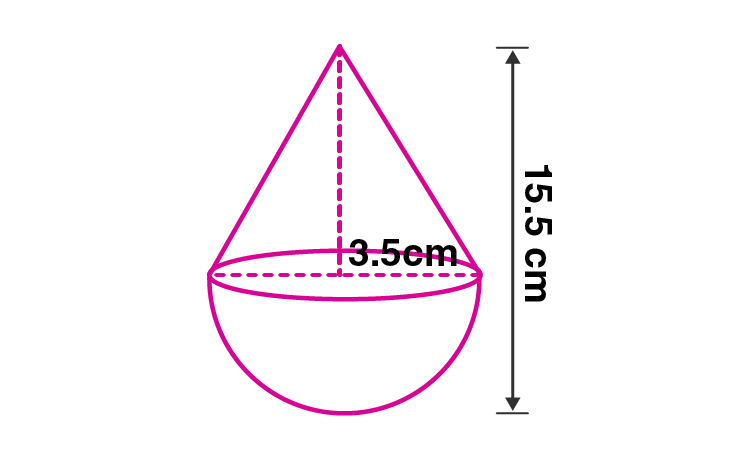Newbie

# A toy is in the form of a cone of radius 3.5 cm mounted on a hemisphere of same radius. If the total height of the toy is 15.5 cm, find the total surface area of the toy.

• 0

This is the Important question of class 10 Based on Mensuration Chapter of M.L Aggarwal book for ICSE BOARD.
Here a toy is in the form of a cone whose radius is given.It is mounted on a hemisphere of same radius and the total height of the toy is also given ,then find the total surface area of the toy.
This is the Question Number 09, Exercise 17.4 of M.L Aggarwal.

Share

1.Given radius of the cone, r = 3.5 cm

Radius of hemisphere, r = 3.5 cm

Total height of the toy = 15.5 cm

Height of the cone = 15.5 – 3.5 = 12 cm

Slant height of the cone, = √(h2+r2)

= √(122+3.52)

= √(144+12.25)

= √(156.25)

= 12.5 cm

Total surface area of the toy = curved surface area of cone + curved surface area of the hemisphere

= rl + 2r2

= r(l+2r)

= (22/7)×3.5×(12.5+2×3.5)

= (77/7)×(12.5+7)

= 11×19.5

= 214.5 cm2

Hence the total surface area of the toy is 214.5 cm2.

• 0